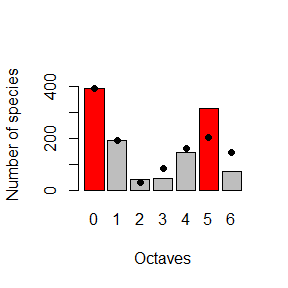# Overview

The gambin distribution is a sample distribution based on a stochastic model of species abundances, and has been demonstrated to fit empirical data better than the most commonly used species-abundance distribution (SAD) models (see Matthews et al. (2014) and Ugland et al. (2007)). Gambin is a stochastic model which combines the gamma distribution with a binomial sampling method. To fit the gambin distribution, the abundance data is first binned into octaves using a simple log2 transform that doubles the number of abundance classes within each octave. Thus, octave 0 contains the number of species with 1 individual, octave 1 the number of species with 2 or 3 individuals, octave 2 the number of species with 4 to 7 individuals, and so forth (method 3 in Gray, Bjorgesaeter, and Ugland (2006)).

The gambin distribution is flexible, meaning it can fit a variety of empirical SAD shapes (including lognormal and logseries-like shapes), and that the distribution shape (in the context of the unimodal gambin model) is adequately characterised by the model’s single parameter (α): low values of alpha indicate logserieslike SADs, and high alpha values indicate lognormal-like SADs. As such, the alpha parameter can be used as a metric to compare the shape of SADs from different ecological communities; for example, along an environmental gradient (e.g Arellano et al. (2017))

The expected abundance octave of a species is given by the number of successfull consecutive Bernoulli trials with a given parameter $$p$$. The parameter $$p$$ of species is assumed to distributed according to a gamma distribution. This approach can be viewed as linking the gamma distribution with the probability of success in a binomial process with $$x$$ trials. Use the fit_abundances() function to fit the gambin model to a vector of species abundances, optionally using a subsample of the individuals. The package estimates the alpha (shape) parameter with associated confidence intervals. Methods are provided for plotting the results, and for calculating the likelihood of fits. The summary() function provides the confidence intervals around alpha, and also the results of a X2 goodness of fit test. Prior to package version 2.4.4, we simply used the default degrees of freedom in this test (i.e. number of data points - 1). This is not optimal as the degrees of freedom should arguably also include the number of parameters used to fit the gambin model itself. As such, in version 2.4.4 we have edited the degrees of freedom to reflect this. One problem is that the chisq.test() function in R does not have an argument for setting the degrees of freedom; thus, we have had to use a workaround. As a result of this change, X2 results generated using older versions of the package will differ slightly from those using 2.4.4 and later.

It has become increasingly apparent that many empirical SADs are in fact multimodal (Antao et al. (2017)). As such, recent work has focused on expanding the standard unimodal gambin model to allow it to fit distributions with multiple modes (Matthews et al. (2019)). For example, the bimodal gambin model can be calculated as the integration of two gambin distributions. The corresponding likelihood function for the bimodal gambin model contain’s four parameters: the shape parameters for the first and second group, the max octave of the first group (as this is allowed to vary), and one splitting parameter (split) representing the fraction of objects in the first group.It is relatively straightforward to extend the above approach for fitting the bimodal gambin model by maximum likelihood, to fitting gambin models with g modes. For each additional mode, a further three parameters are needed: the additional alpha, max octave and split parameters (see Matthews et al. (2019)). Use the fit_abundances() function in combination with the no_of_components of argument. The default is no_of_components = 1, which fits the standard unimodal gambin model. no_of_components = 2, fits the bimodal gambin model, and so on. As the optimisation procedure takes long with no_of_components > 1, it is possible to use the cores argument within fit_abundances to make use of parallel processing in the maximum likelihood optimisation.

The deconstruct_modes() function can then be used to examine a multimodal gambin model fit. The function provides the location of the modal octaves of each component distribution and (if species classification data are provided) determines the proportion of different types of species in each octave.

Often the aim of SAD studies is to compare the form of the SAD across different sites / samples. The alpha parameter of the one component gambin model (alpha) has been found to provide a useful metric in this regard. Use the mult_abundances() function to calculate alpha values for a set of different samples / sites. However, because the alpha parameter of the gambin model is dependent on sample size, when comparing the alpha values between sites it can be useful to first standardise the number of individuals in all sites. By default, the mult_abundances() function calculates the total number of individuals in each site and selects the minimum value for standardising. This minimum number of individuals is then sampled from each site and the gambin model fitted to this subsample and the alpha value stored. This process is then repeated N times and the mean alpha value is calculated for each site.

## Example

library("gambin")
data(moths, package="gambin")

##unimodal model
fit = fit_abundances(moths)
fit$alpha ##  1.644694 barplot(fit) points(fit)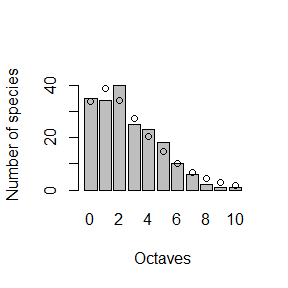AIC(fit) ##  803.6409 ##unimodal model (fit to a subsample of 1000 individuals) fit2 = fit_abundances(moths, subsample = 1000) fit2$alpha
##  0.8904631
barplot(fit2)
points(fit2)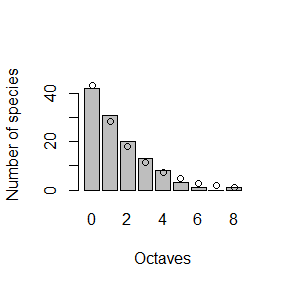AIC(fit2)
##  394.7729
##bimodal model (using 3 cores)

#simulate bimodal gambin distribution
x1 = rgambin(600, 5, 10)
x2 = rgambin(300, 1, 10)
x = table(c(x1,x2))
freq = as.vector(x)
values = as.numeric(as.character(names(x)))
abundances = data.frame(octave=values, species = freq)

#fit bimodal model to simulated data
fit3 = fit_abundances(abundances, no_of_components = 2, cores = 1)
## Using 1 core. Your machine has 12 available.
barplot(fit3)
points(fit3)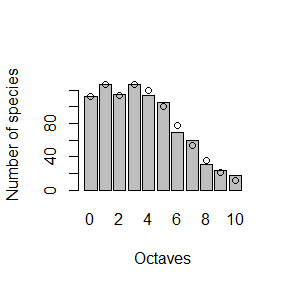AIC(fit3)
##  4062.259
#compare with AIC of unimodal model
AIC(fit_abundances(abundances))
##  4087.551
#fit a bimodal model to a species classification dataset
#and calculate the number of the differet categories in each octave
data(categ, package="gambin")
fits2 = fit_abundances(categ\$abundances, no_of_components = 2)
## Using 1 core. Your machine has 12 available.
d1 <- deconstruct_modes(fits2, dat = categ, peak_val = NULL, abundances = "abundances",
species = "species", categ = "status", col.statu = c("green", "red", "blue"),
plot_legend = FALSE)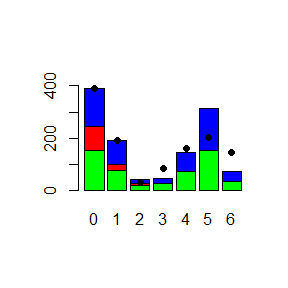#do the same but don't provide category data - this just highlights the modal octaves
d2 <- deconstruct_modes(fits2, dat = categ, peak_val = NULL, abundances = "abundances",
species = "species", categ = NULL)